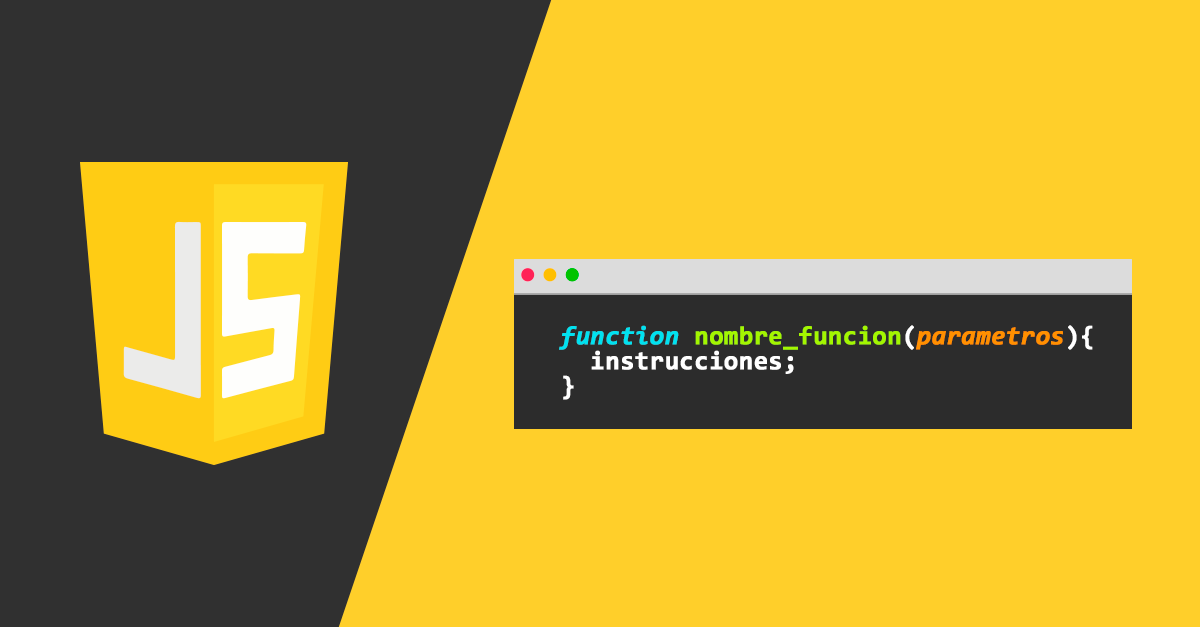September 20, 2019 - 5:53 pm

# The Fundamentals of JavaScript (Part 2)

After a short break, here I am writing the second part of the fundamentals of JavaScript. In this part, we are going to discuss operators, loop,  conditions. Let’s get started.

#### Operators

We know many operators from school like “+”, “-”, “/”, “*” those functionalities the same as before.

#### Assignment (=)

Let’s note that an assignment = is also an operator. It is listed in the precedence table with the very low priority of 3. That is why when we assign a value to a variable, like x = 2 + 2 + 1, first, calculate the equation, assign a value to x variable.

#### Reminder(%)

The remainder operator %, despite its appearance, is not related to percents. The result of a % b is the remainder of the integer division of a by b. For example :

#### Exponentiation(**)

The exponentiation operator ** is a recent addition to the language. For a natural number b, the result of a ** b is multiplied by itself b times. For example :

#### Increment/Decrement

Increment ++  increases a variable by 1

let counter = 2;
counter++;
alert( counter ) // Value 3;
Decrement  — decreases a variable by 1
let counter = 2;
Counter–;
alert( counter ) // Value 1;

• AND (&&)
• OR(||)

#### Conditional Operators

• If statement

The if(…) statement evaluates a condition in parentheses and, if the result is true, executes a block of code.
For example :

In this above example, the condition is a simple equality check (year == 2019). Then the alert year 2019.

• Boolean Conversion
1. A number 0, an empty string ” “, null, undefined, and NaN all become false.
2. Other values become true, so they are called “truthy”.
• The else Clause

The if statement may contain an optional “else” block. It executes when the condition is false.

• Several conditions: else if

Sometimes, we’d like to test several variants of a condition. The else if clause lets us do that.

#### Loops: While and For

• The while loop:

The while loop has the following syntax

While the condition is true the code from the loop body will execute

After every execution of the loop, the value of i will increase by 1, when i become 3, it will not execute code inside while body.

• do…while loop

The condition check is executed after the loop executes the first time. A better example of do…while loop is a bank atm machine.

For example:

This form of syntax should be used when we want the body of the loop to execute at least once.